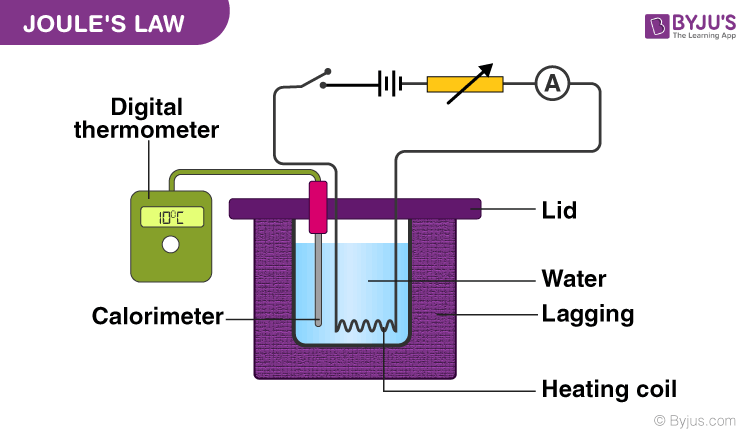# Joule's Law

We are aware of the heating impact of electric current. The heat is produced due to the collision electrons in the wire. You might have wondered about the amount of heat generated during the flow of current through a wire and the parameters and conditions it is based upon. To answer all these questions, Joule gave a formula that describes this phenomenon precisely and called it Joule’s Law.

## Joule’s Law of Heating

Joule’s law is a mathematical description of the rate at which resistance in a circuit converts electric energy into heat energy.The English physicist James Prescott discovered that the amount of heat per second that develops in a current-carrying conductor is proportional to the electrical resistance of the wire and the square of the current.

The heat that is generated because of the current flow in an electric wire is described in Joules. The mathematical expression of Joule’s law is as explained below.

### Joule’s first law

The joule’s first law shows the relationship between heat produced by flowing electric current through a conductor.

Q = I2 R T

Where,

• Q indicates the amount of heat
• I show electric current
• R is the amount of electric resistance in the conductor
• T denotes time
• The amount of generated heat is proportional to the wire’s electrical resistance when the current in the circuit and the flow of current is not changed.
• The amount of generated heat in a conductor carrying current is proportional to the square of the current flow through the circuit when the electrical resistance and current supply is constant.
• The amount of heat produced because of the current flow is proportional to the time of flow when the resistance and current flow is kept constant.

### Solved Example

Q1) Calculate the heat energy produced in resistance of 5 Ω when 3 A current flows through it for 2 minutes.
The amount of heat produced by the conductor is given by the formula:
Q = I2 R T
Substituting the values in the above equation we get
Q = 32× 5 × 2 × 60 = 5400 J

Q2) An heater of resistance 300 Ω is connected to the main supply for 30 mins. If 10 A current flows through the filament of the heater then what is the heat produced in the heater?
The amount of heat produced by the heater is calculated as follows:
Q=I2RT
substituting the values in the equation, we get
Q = 102 × 300 × 30 × 60 = 54000000 J or 54 MJ.

To know more about Joule’s law of thermodynamics and solved examples, you can visit us BYJU’S.

Stay tuned to BYJU’S and Fall in Love with Learning!

Test your knowledge on Joules law